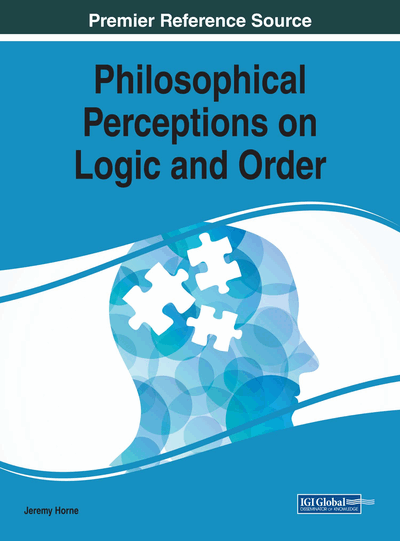# Extensions and Limitations to Logic

Thomas J. Marlowe (Seton Hall University, USA)
DOI: 10.4018/978-1-5225-2443-4.ch005
Available
\$37.50
No Current Special Offers

## Abstract

Classical (Aristotelean or Boolean) logics provide a solid foundation for mathematical reasoning, but are limited in expressivity and necessarily incomplete. Effective understanding of logic in the modern world entails for the instructor and advanced students an understanding of the wider context. This chapter surveys standard extensions used in mathematical reasoning, artificial intelligence and cognitive science, and natural language reasoning and understanding, as well as inherent limitations on reasoning and computing. Initial technical extensions include equality of terms, integer arithmetic and quantification over sets and relations. To deal with natural reasoning, the chapter explores temporal and modal logics, fuzzy logic and probabilistic models, and relevance logic. Finally, the chapter considers limitations to logic and knowledge, via an overview of the fundamental results of Turing, Gödel, and others, and their connection to the state of mathematics, computing and science in the modern world.
Chapter Preview
Top

## Introduction

### The Problem

From at least the time of Leibniz (Ishiguro, 1990; Kemerling, 2011; Shabel, 2005), logicians and mathematicians have had a dream of representing both information and thought in logic, and using that to deduce the truth and resolve conflicts. In line with attempts by Frege (Frege, 1950; Wikipedia, n.d.f)1 and Russell & Whitehead (Whitehead & Russell, 1912; Wikipedia, n.d.p) to create complete logical models for mathematics (Demopoulos & Clark, 2005; Franzén, 2005), David Hilbert in his 1900 address to the International Conference of Mathematicians in Paris (Hilbert, 1900) challenged mathematicians with a list of 23 problems (Browder, 1976). For this chapter, the most relevant are the Second Problem, asking whether arithmetic could be proved consistent, and the Tenth Problem, asking for a general solution technique for Diophantine equations. (See (Hawking, 2007) for a discussion of and excerpts from the work of Diophantus of Alexandria, who first studied the class of equations now known as Diophantine equations.) Hilbert’s First Problem, on the Continuum Hypothesis (Martin, 1976), is also mentioned in Applications of Modal Logic, below.

By the middle of the 20th century, however, it had become clear:

• That constructs such as “the set of all sets” lead to a contradiction (“Russell’s Paradox”) (Ellenberg, 2015; Stanford Encyclopedia of Philosophy, 2014)—see Undecidability and Rice’s Theorem, below;

• That even physical information is inherently imprecise (the Heisenberg Principle (Eisberg, 1961; Hilgevoord & Uffink, 2016; Perry, 2012) and dependent on the observer’s frame of reference (relativity (Wald, 2010));

• That quantifying cause-and-effect may rely on arbitrarily precise representation of information (chaos theory (Gleick, 2011)), may undergo rapid or even instantaneous transitions to different response regimes (for example, catastrophe theory (Poston & Stewart, 2014)), and may entail probabilistically random events (for example, atomic decays or random mutations) (Acín, 2014; Bryn, 2015);

• That statistical information is needed in discussing human reactions and characteristics, unless a very large number of independent factors can be observed and encoded, and possibly not even then—part of the motivation behind Big Data, for which see for example (Moorthy et al., 2015);

• And that, perhaps most surprisingly, there are inherent limitations to logic and computation, so that the Leibniz program can never be realized in its entirety, even in principle.

Due to these limitations, and also for several other major purposes—to understand human cognition and reasoning, to develop artificial intelligence/expert systems to assist in problem solving (and because it is fun) (Wikipedia, n.d.o), and to explore the boundaries of mathematical and logical reasoning—the past 200 years have seen a renewed interest in both the foundations and the frontiers of logic and its relatives, mathematics and computer science.

The significance of such studies has only increased with the pervasiveness and ubiquity of computation in the modern world, impacted by not only the practice but also the theory of computing (Copeland, Posy & Shagrir, 2013). Below, we consider at some of those frontiers, and in particular several extensions and limitations.

## Complete Chapter List

Search this Book:
Reset